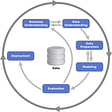# Introduction

Anomaly-based detection usually uses statistics from a large number of packets. It should define what is normal and take into account a deviation from that normal behaviour. Zeek is a leading anomaly-based IDS (Intrusion Detection System) that reads all traffic passing through the network and generates quite a number of logs in tab-delimited columns. You can find more information on Zeek Logs page.

Although supervised ML (Machine Learning) techniques work to produce good results, they always require a training dataset that is often not readily available. Therefore, in this tutorial, we choose a clustering-based unsupervised approach to detect anomalous DNS traffic. With the Python library Zeek Analysis Toolkit (ZAT), you can extract data formats that can be read by ML packages from raw Zeek log files. Instead of capturing data from a live network, we will explore PCAP traffic.

# What is Zeek?

Zeek is a network monitoring framework designed to analyze complex, high-throughput networks. It collects more than 400 data fields in more than 30 protocols. Moreover, Zeek allows us to write our own custom scripts (See anomalous-dns). Zeek is designed to provide a better source of network data for threat hunting and incident response. It produces various timestamped log files synchronized with a unique ID

# Analyzing Zeek Log Files

Using ZAT libraries, we have converted Zeek logs to a Pandas DataFrame. Pandas is an open source Python library that provides useful data structures and data analysis tools. We use Jupyter notebooks to develop our ML applications in an interactive computing environment.

We create a Pandas dataframe from the Zeek DNS log and select some useful features to utilize. Besides using these selected features, we calculate query length and entropy.

`log_to_df = LogToDataFrame()dns_df = log_to_df.create_dataframe('./zeek_logs/dns.log')features = ['id.resp_h', 'Z', 'proto', 'qtype_name', 'query_length', 'answer_length', 'entropy']dns_df[features].head()`

Before we start to apply machine learning, we need to process both numerical and categorical data in our dataframe. One-hot encoding is a way to convert numeric and categorical data types into binary files and then combine them. Categorical data are variables that contain label values, and are also called nominal. Although some algorithms can directly use categorical data such as decision trees without transforming them, many algorithms require all input variables and output variables to be numeric. ZAT scikit-learn transformer class (DataFrameToMatrix) can convert the Pandas DataFrame to an numpy (numerical python) array matrix.

`to_matrix = DataFrameToMatrix()zeek_matrix = to_matrix.fit_transform(dns_df[features], normalize=True)`

Now, we are ready to use ML Algorithms with scikit-learn.

Isolation Forest algorithm is used to return the anomaly score for each record. It isolates observations by randomly selecting a split value between the maximum and minimum values of the selected feature in a recursive manner. Let’s `Train/fit` and `predict` anomalous instances using the Isolation Forest model.

`odd_clf = IsolationForest(contamination=0.25)odd_clf.fit(zeek_matrix)odd_df = dns_df[features][odd_clf.predict(zeek_matrix) == -1]odd_df.head()`

After the isolation forest algorithm, a new dataframe with the same features is created.

Before applying K-Means clustering, we are going to apply one-hot encoding again and the PCA algorithm. We employ PCA to reduce the number of features in our data set, and then generate a new dataframe from our K-means clustering results. K-Means clustering is to find K groups with similar patterns in the data, also called distance-based algorithm. K-means also refers to averaging of records.

`odd_matrix = to_matrix.fit_transform(odd_df)kmeans = KMeans(n_clusters=4).fit_predict(odd_matrix)pca = PCA(n_components=3).fit_transform(odd_matrix)odd_df['x'] = pca[:, 0] # PCA X Columnodd_df['y'] = pca[:, 1] # PCA Y Columnodd_df['cluster'] = kmeansodd_df.head()`

Let’s create ZAT-based graphs in `matplotlib` and generate the cluster groups to see anomalous.

The outliers in anomaly detection are considered much smaller than the whole dataset, and don’t follow the overall trend. For instance, Cluster-1 has undefined values for certain features instead of having numeric data, and Cluster-3 has the Z flag as 1, which should be 0.

# Supervised Zeek Anomaly detector

The zeek_anomaly_detector is an anomaly detector for Zeek’s `conn.log` files using ZAT and PyOD, a python toolkit to detect outlying objects. The script first creates a Pandas dataframe from the log, and sanitizes the data for better performance. The selected features are numbers only, so there is no need to apply one-code encoding.

`features = ['duration', 'orig_bytes', 'id.resp_p', 'resp_bytes', 'orig_ip_bytes', 'resp_pkts', 'resp_ip_bytes']`

The anomaly detector script offers outlier detection techniques (ODT) to detect anomalous observations. In the outlier detection, the training dataset is considered contaminated with outliers. Detection predicts the most intense observations. The algorithm calculates a score by looking at observations with lower density than its neighbours.

PyOD (Python Outlier Detection) is the most comprehensive and scalable open-source Python toolkit for detecting anomalies and provides over 40 outlier detection algorithms, see the algorithm list. The Zeek anomaly detector uses the PyOD library to apply `predict` method to tag the outliers, and `decision_function` to score them.

`# Fit the model to the train dataestimator.fit(X_train)# get the prediction on the test datay_test_pred = estimator.predict(X_test) # outlier labels (0 or 1)y_test_scores = estimator.decision_function(X_test) # outlier scores`

Let’s create a table including the predicted top anomalies.

# Conclusions

Although anomaly detection can be done by supervised techniques, in most cases, unsupervised learning is preferred. We propose an unsupervised machine learning approach that combines the K-Means algorithm with the Isolation Forest.

Feature selection plays an important role in improving outlier detection. High-dimensional features create difficulties for unsupervised algorithms, so feature selection methods have become widely used.

ZAT is a great python package that supports processing and analysis of Zeek data with Pandas and Scikit-learn. PyOD includes more than 40 detection algorithms for detecting outlying objects.

--

--

--

## More from Hootsuite Engineering

Hootsuite's Engineering Blog

## BEIT: BERT Pre-Training of Image Transformers## AI, ML, DL and Everything in Between## Selecting the Right Scoring Pattern for Machine Learning## Development of social chatbots## Using supervised machine learning to quantify political rhetoric## Need help hitting your word count?## Machine Learning Tools in OpenSource vs Cloud## Overcome Imbalance in your datasets — PART II## Building Highly Accurate Fraud Detection System## AI Models in Production — The Beginning of the end